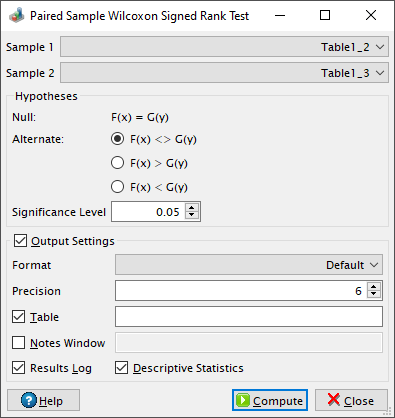# Paired Sample Wilcoxon Signed Rank Test Dialog

This dialog is activated by selecting the Paired Sample Wilcoxon Signed Rank Test... command from the Statistics -> Nonparametric Tests -> menu. It can be used in order to determine whether two dependent samples were selected from populations having the same distribution. The test procedure is explained in a Wikipedia article. If the reduced sample size, `Nr`, after excluding the ties, is more than 200, QtiPlot uses the normal distribution approximation in order to calculate a p-value and to take the decision of rejecting or not the null hypothesis. Bellow this threshold QtiPlot also uses an exact method to compute the p-values using the algorithm explained by Stenver Jerkku in his Bachelor's thesis, Parallel Wilcoxon signed-rank tests, 2014, University of Tartu, Institute of Computer Science, chapter 2.7.2, page 18. The null hypothesis is rejected if the probability is lower than the value of the significance level.

Figure 5-123. The paired sample Wilcoxon signed rank test dialog.QtiPlot also computes the test statistic, W, and a Z-score, using the formula: Z = [W-0.5sign(W)]/Var1/2, where 0.5 is a continuity correction factor and the variance is Var = Nr(Nr + 1)(2*Nr + 1)/6.

If there are missing values in any of the paired source data columns, the whole pair will be excluded from the analysis.